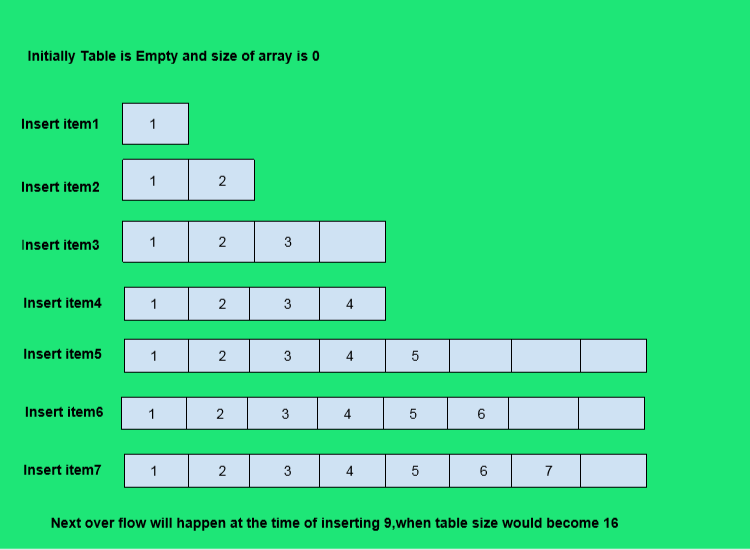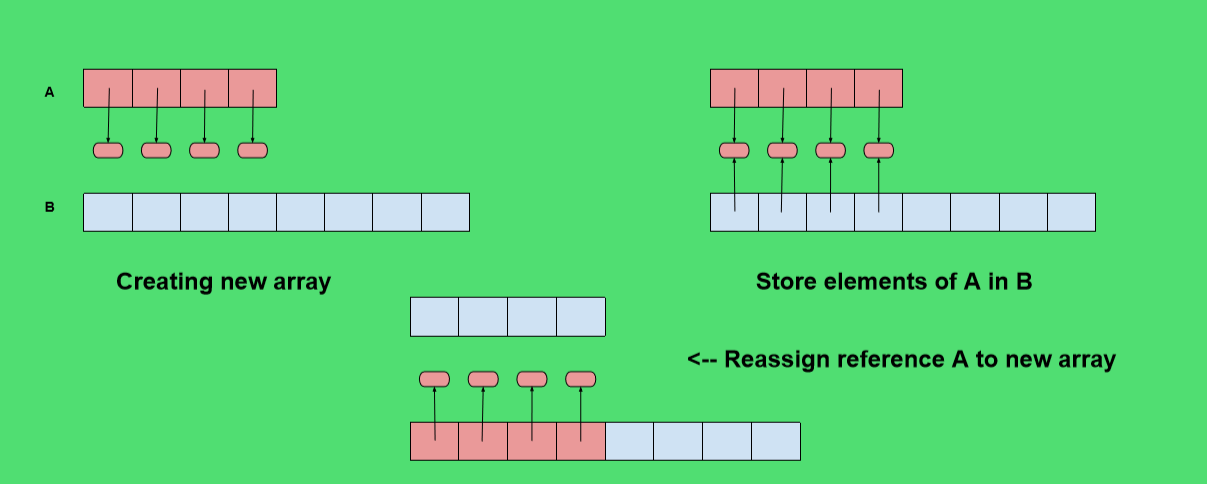# Implementation of Dynamic Array in Python

• Difficulty Level : Basic
• Last Updated : 19 Aug, 2022

What is a dynamic array?

A dynamic array is similar to an array, but with the difference that its size can be dynamically modified at runtime. Don’t need to specify how much large an array beforehand. The elements of an array occupy a contiguous block of memory, and once created, its size cannot be changed. A dynamic array can, once the array is filled, allocate a bigger chunk of memory, copy the contents from the original array to this new space, and continue to fill the available slots.We’ll be using a built in library called ctypes of python . Check out the documentation for more info, but its basically going to be used here as a raw array from the ctypes module.

A quick note on public vs private methods, we can use an underscore _ before the method name to keep it non-public.

For example:

## Python

 `class` `M(``object``):``    ` `    ``def` `public(``self``):``        ``print` `'Use Tab to see me !'``        ` `    ``def` `_private(``self``):``        ``print` `"You won't be able to Tab to see me !"`

## Python

 `m ``=` `M()``m.public()`

Output:

`Use Tab to see me!`

## Python

 `m._private()`

Output:

`You won't be able to see me!`

Dynamic Array Logic Implementation:

The key is to provide means to grows an array A that stores the elements of a list. We can’t actually grow the array, its capacity is fixed. If an element is appended to a list at a time, when the underlying array is full, we need to perform following steps.

1. Allocate a new array B with larger capacity (A commonly used rule for the new array is to have twice the capacity of the existing array )
2. Set B[i]=A[i], for i=0 to n-1 where n denotes the current no of items.
3. Set A=B that is, we hence forth use B as the array of supporting list.
4. Insert new element in the new array.Dynamic Array Code Implementation:

## Python

 `import` `ctypes` `class` `DynamicArray(``object``):``    ``'''``    ``DYNAMIC ARRAY CLASS (Similar to Python List)``    ``'''``    ` `    ``def` `__init__(``self``):``        ``self``.n ``=` `0` `# Count actual elements (Default is 0)``        ``self``.capacity ``=` `1` `# Default Capacity``        ``self``.A ``=` `self``.make_array(``self``.capacity)``        ` `    ``def` `__len__(``self``):``        ``"""``        ``Return number of elements sorted in array``        ``"""``        ``return` `self``.n``    ` `    ``def` `__getitem__(``self``, k):``        ``"""``        ``Return element at index k``        ``"""``        ``if` `not` `0` `<``=` `k <``self``.n:``            ``# Check it k index is in bounds of array``            ``return` `IndexError(``'K is out of bounds !'``)``        ` `        ``return` `self``.A[k] ``# Retrieve from the array at index k``        ` `    ``def` `append(``self``, ele):``        ``"""``        ``Add element to end of the array``        ``"""``        ``if` `self``.n ``=``=` `self``.capacity:``            ``# Double capacity if not enough room``            ``self``._resize(``2` `*` `self``.capacity)``        ` `        ``self``.A[``self``.n] ``=` `ele ``# Set self.n index to element``        ``self``.n ``+``=` `1` `    ``def` `insertAt(``self``,item,index):``        ``"""``        ``This function inserts the item at any specified index.``        ``"""` `        ` `        ``if` `index<``0` `or` `index>``self``.n:``            ``print``(``"please enter appropriate index.."``)``            ``return``        ` `        ``if` `self``.n``=``=``self``.capacity:``            ``self``._resize(``2``*``self``.capacity)``            ` `        ` `        ``for` `i ``in` `range``(``self``.n``-``1``,index``-``1``,``-``1``):``            ``self``.A[i``+``1``]``=``self``.A[i]``            ` `        ` `        ``self``.A[index]``=``item``        ``self``.n``+``=``1`  `        ` `    ``def` `delete(``self``):``        ``"""``        ``This function deletes item from the end of array``        ``"""` `        ``if` `self``.n``=``=``0``:``            ``print``(``"Array is empty deletion not Possible"``)``            ``return``        ` `        ``self``.A[``self``.n``-``1``]``=``0``        ``self``.n``-``=``1``        ` `        ` `        ` `    ` `    ``def` `removeAt(``self``,index):``        ``"""``        ``This function deletes item from a specified index..``        ``"""`        `        ``if` `self``.n``=``=``0``:``            ``print``(``"Array is empty deletion not Possible"``)``            ``return``                ` `        ``if` `index<``0` `or` `index>``=``self``.n:``            ``return` `IndexError(``"Index out of bound....deletion not possible"``)       ``        ` `        ``if` `index``=``=``self``.n``-``1``:``            ``self``.A[index]``=``0``            ``self``.n``-``=``1``            ``return`       `        ` `        ``for` `i ``in` `range``(index,``self``.n``-``1``):``            ``self``.A[i]``=``self``.A[i``+``1``]           ``            ` `        ` `        ``self``.A[``self``.n``-``1``]``=``0``        ``self``.n``-``=``1` `        ` `    ``def` `_resize(``self``, new_cap):``        ``"""``        ``Resize internal array to capacity new_cap``        ``"""``        ` `        ``B ``=` `self``.make_array(new_cap) ``# New bigger array``        ` `        ``for` `k ``in` `range``(``self``.n): ``# Reference all existing values``            ``B[k] ``=` `self``.A[k]``            ` `        ``self``.A ``=` `B ``# Call A the new bigger array``        ``self``.capacity ``=` `new_cap ``# Reset the capacity``        ` `    ``def` `make_array(``self``, new_cap):``        ``"""``        ``Returns a new array with new_cap capacity``        ``"""``        ``return` `(new_cap ``*` `ctypes.py_object)()`

## Python

 `# Instantiate``arr ``=` `DynamicArray()``# Append new element``arr.append(``1``)``len``(arr)`

Output:

`1`

## Python

 `# Append new element``arr.append(``2``)``# Check length``len``(arr)`

Output:

`2`

## Python

 `# Index``arr[``0``]`

Output:

`1`

## Python

 `arr[``1``]`

Output:

`2`

Awesome, we made our own dynamic array! Play around with it and see how it auto-resizes.

My Personal Notes arrow_drop_up